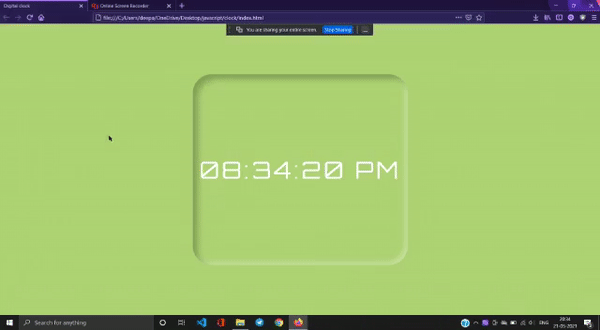# Design a Digital Clock in Neumorphism Style using JavaScript

• Last Updated : 09 Jul, 2021

A clock is a device that used to measure time. Clocks are a useful element for any UI if used in a proper way. Clocks can be used in sites where time is the main concern like some booking sites or some app showing arriving times of trains, buses, flights, etc. Clock is basically of two types, Analog and Digital. Here, we will design the digital clock and add some styling to make it more attractive.

Approach: The approach is to use the date object to get time on every second and then re-rendering time on the browser using the new time that we got by calling the same function each second and to make clocks looks like more attractive.

HTML & CSS Code: In this section, we have a dummy time in the format of “HH:MM:SS” wrapped inside a “div” tag and we have included the CSS and JavaScript file externally.

## HTML

 ```<``html``>` `<``head``>``    ``<``title``>Digital clock` `    ``<``style``>` `        ``/* Link for Google font */``        ``@import url(``'https://fonts.googleapis.com/css2?family=Orbitron&display=swap');` `        ``body {` `            ``/* Set background color of body */``            ``background-color: #afd275;` `            ``/* Place item to center */``            ``align-items: center;``            ``display: flex;``            ``justify-content: center;` `            ``/* Specify the vertical height */``            ``height: 100vh;``            ``overflow-y: hidden;``        ``}` `        ``.clock {``            ``position: absolute;` `            ``/* Put the clock content on``            ``center of the screen */``            ``top: 50%;``            ``left: 50%;``            ``transform: translateX(-50%) translateY(-50%);``            ``color: white;``            ``font-size: 60px;``            ``font-family: Orbitron;` `            ``/* Provide space between letter of clock */``            ``letter-spacing: 7px;``            ``align-items: center;``            ``border-radius: 50px;``            ``display: flex;``            ``justify-content: center;``            ``margin-right: 4rem;``            ``height: 500px;``            ``width: 550px;` `            ``/* Set the neumorphism effect to``             ``the body of clock */``            ``background-color: #afd275;``            ``box-shadow: inset 12px 12px 16px 0 rgba(0, 0, 0, 0.25),``                ``inset -8px -8px 12px 0 rgba(255, 255, 255, 0.3);``        ``}``    ````` `<``body``>``    ``<``div` `class``=``"container"``>``        ``<``div` `id``=``"MyClockDisplay"``            ``class``=``"clock"` `onload``=``"showTime()"``>``        ````    ``` `    ````    ``<``script` `src``=``"index.js"``>``` ``

JavaScript Code:

• Step 1: Create a function “showTime”.
• Step 2: Create an instance of the Date object.
• Step 3: Using the methods of Date object get “hours”, “minute”, and “seconds”.
• Step 4: Set AM/PM depending on the hour value. The Date object works in the 24-hour format so we change the hour back to 1 when it gets larger than 12. The AM/PM also changes according to that.
• Step 5: Now make a string using the same HH:MM:SS format changing the hour, minute, and, second value with the values, we get from Date object methods.
• Step 6: Now replace the string variable in the “div” using innerHTML property.
• Step 7: To call the function every second use setInterval() method and set time-interval as 1000ms which is equal to 1s.
• Step 8: Now call the function at the end to start function at exact reloading/rendering time as setInterval() will call first after 1s of rendering.

## Javascript

 `// Function to display the time``function` `showTime() {` `    ``// Using Date object to get``    ``// today's date and time``    ``var` `date = ``new` `Date();` `    ``// getHOurs() function is used``    ``// to get hours ones``    ``var` `h = date.getHours(); ``// 0 - 23` `    ``// getMinutes() function is``    ``// used to get minutes ones``    ``var` `m = date.getMinutes(); ``// 0 - 59` `    ``// getSecond() function is``    ``// used to get seconds ones``    ``var` `s = date.getSeconds(); ``// 0 - 59` `    ``// To show am or pm``    ``var` `session = ``"AM"``;` `    ``// Condition to check that if hours``    ``// reaches to 12 then it again start``    ``// with 12``    ``if` `(h == 0) {``        ``h = 12;``    ``}` `    ``// If hour exceeds 12 than it will``    ``// be subtract from 12 and make``    ``// session as pm``    ``if` `(h > 12) {``        ``h = h - 12;``        ``session = ``"PM"``;``    ``}` `    ``h = (h < 10) ? ``"0"` `+ h : h;``    ``m = (m < 10) ? ``"0"` `+ m : m;``    ``s = (s < 10) ? ``"0"` `+ s : s;` `    ``var` `time = h + ``":"` `+ m + ``":"``            ``+ s + ``" "` `+ session;` `    ``// Using DOM element to display``    ``// elements on screen``    ``document.getElementById(``"MyClockDisplay"``)``                ``.innerText = time;` `    ``document.getElementById(``"MyClockDisplay"``)``                ``.textContent = time;` `    ``// Call the function every second use``    ``// setInterval() method and set time-interval``    ``// as 1000ms which is equal to 1s``    ``setTimeout(showTime, 1000);``}` `showTime();`

Output:My Personal Notes arrow_drop_up